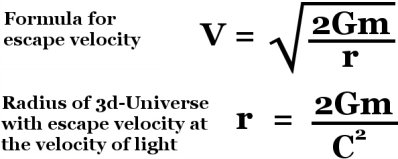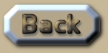Volume of the Universe

 Volume of the Universe The shape of the Universe has been established as a 3d-hyper-surface of a 4d-hyper-sphere. But if we are dealing with a 1d-Universe on the edge of a 2d-disc, or instead of this we also consider flatland: a 2d-Universe on the surface of a 3d-sphere; then the ratio between the diameter and the circumference is still pi. So it stands to reason that this same ratio of pi is still perfectly good for a 4d-hypersphere. However when we look back to the origin of the Universe, we are not looking back directly along the radius. We are looking outwards, and then back. Consider this diagram very slowly and carefully: .
 The inhabitants of a disc-Universe live on the 1-dimensional edge. If the light could travel directly along the radius of expansion, they would see themselves in the past. But instead they see objects a distance away from them on the expanding edge. So the light cannot travel directly from the centre to the edge along the radius of expansion. This may be difficult to comprehend at first, but once you see it, then it should be fairly easy to conclude from this premise that: The radius is thus 13.7 billion light-years divided by half of pi: Radius = 8.72 billion light-years or 8.26 x 10^25 meters. Calculating the volume is a bit trickier because we need the formula for the 3d-hyper-surface of a 4d-hyper-sphere. Of course the 4d-hyper-volume is not the same as this because that would be a measurement in m^4, whereas a 3d measurement results in m^3. .
 This is also not the same as a simple 3d-volume either because our Universe has no visible 3d-edge to it like all 3d-volumes do actually have. So the volume of the Universe is 1.1x10^79 m^3 or 1.1x10^70 km^3: .
 Although, some would claim that 4d-space is an untenable idea. So is it meaningful to postulate the possibility that space is just ordinary 3d-space? In this sense, the galaxies would all be receding from our current position into an endless void. In this scenario, all the mass in the Universe (3x10^52kg) could have at some point been contained in a small spherical volume of space smaller than the distance to the nearest star. At this point it would have had the average density of water. But then the escape velocity would have been 70 000 times the velocity of light. The obvious problem here is that such a theory would not be able to allow for the Relativistic notion that velocity cannot exceed the velocity of light. But what would the radius of the mass of the Universe need to be, such that its escape velocity is equal to the velocity of light? .And it is quite a surprise to find that the mass of the Universe would need to be 4.7 billion light-years in radius to have an escape velocity of the speed of light. If you are a die-hard Relativist may need to think about that in the context of how you have conceptualized the Universe when it was less than 4.7 billion-light-years in radius. .This is an extract summary of Chapter XXX of the book: Flight Light and Spin